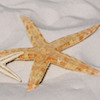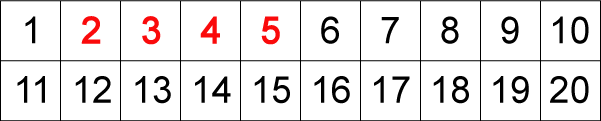#### You may also like### Month Mania

Can you design a new shape for the twenty-eight squares and arrange the numbers in a logical way? What patterns do you notice?### Starfish Spotting

How many starfish could there be on the beach, and how many children, if I can see 28 arms?### Catrina's Cards

How would you find out how many football cards Catrina has collected?

# What's Left?

## What's Left?

Here is a grid showing the numbers $1-20$:Start with the first red number, $2$. Now, look along the top row of the grid to the next number, $3$.

Can you share $3$ exactly between $2$?

If the answer is yes then shade in the $3$ box. If the answer is no, leave it.

Now take the next number, $4$. Can you share $4$ exactly between $2$?

Again, if you can, shade the box with $4$ in it. Carry on doing the same with all the numbers.

Now do the same with the next red number, $3$. Look along the row for the next number, $4$ and shade all the numbers that will share exactly between $3$.

Now try with $4$ and with $5$.

Which numbers do you have left?

### Why do this problem?

This problem will help young learners to come to some understanding of factors and multiples leading to a first introduction to the idea of prime numbers. They will need to look at multiples of $2$, $3$ and $5$ up to $20$. For some children just making the table to use will be a useful exercise.

### Key questions

Do you know the name of the numbers you have coloured in now you have done the sharing by $2$?
Do you know the name of the numbers you have left out now you have done the sharing by $2$?
Which multiplication table will you find these numbers in?
Which numbers have not been coloured in at all?

### Possible extension

Learners could make a grid which goes up to $40$ or even $100$ adding $6$ and $7$ to the "red" numbers.

### Possible support

Suggest starting by making the table to use. Then colour in the numbers counting in $2$s omitting $2$ itself.